Next: The approximating posterior distribution Up: The probabilistic model Previous: The prior of the   Contents

### The prior of the parameters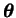Let us denote the elements of the weight matrices of the MLP networks by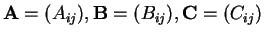and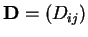. The bias vectors consist similarly of elements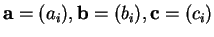and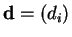.

All the elements of the weight matrices and the bias vectors are assumed to be independent and Gaussian. Their priors are as follows: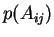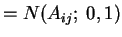(5.27)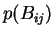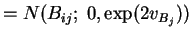(5.28)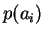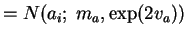(5.29)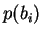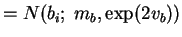(5.30)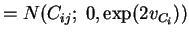(5.31)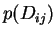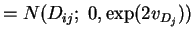(5.32)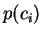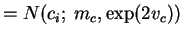(5.33)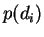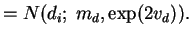(5.34)

These distributions should again be written conditional to the corresponding hyperparameters, but the conditioning variables have been here omitted to keep the notation simpler.

Each of the bias vectors has a hierarchical prior that is shared among the different elements of that particular vector. The hyperparameters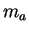,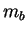,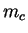,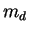,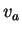,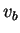,and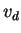all have zero mean Gaussian priors with standard deviation 100, which is a flat, essentially noninformative prior.

The structure of the priors of the weight matrices is much more interesting. The prior of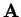is chosen to be fixed to resolve a scaling indeterminacy between the hidden states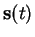and the weights of the MLP networks. This is evident from Equation (5.19) where any scaling in one of these parameters could be compensated by the other without affecting the results in any way. The other weight matricesand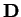have zero mean priors with common variance for all the weights related to a single hidden neuron.

The remaining variance parameters from the priors of the weight matrices and from Equations (5.23), (5.25) and (5.26) again have hierarchical priors defined as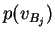(5.35)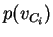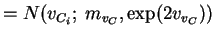(5.36)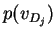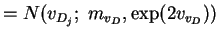(5.37)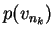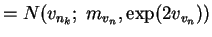(5.38)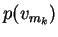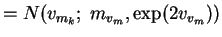(5.39)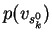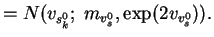(5.40)

The prior distributions of the parameters of these distributions are again zero mean Gaussians with standard deviation 100.Next: The approximating posterior distribution Up: The probabilistic model Previous: The prior of the   Contents
Antti Honkela 2001-05-30